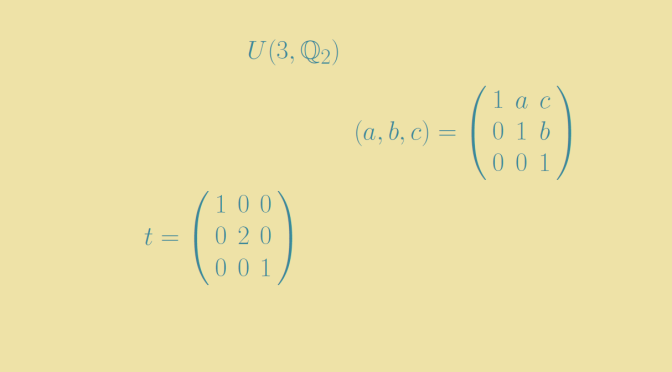# A finitely generated soluble group isomorphic to a proper quotient group

Let $$\mathbb{Q}_2$$ be the ring of rational numbers of the form $$m2^n$$ with $$m, n \in \mathbb{Z}$$ and $$N = U(3, \mathbb{Q}_2)$$ the group of unitriangular matrices of dimension $$3$$ over $$\mathbb{Q}_2$$. Let $$t$$ be the diagonal matrix with diagonal entries: $$1, 2, 1$$ and put $$H = \langle t, N \rangle$$. We will prove that $$H$$ is finitely generated and that one of its quotient group $$G$$ is isomorphic to a proper quotient group of $$G$$.

Denote by $$(a, b, c)$$ the unitriangular matrix $1 + aE_{12} + bE_{23} + cE_{13}=\left( \begin{array}{ccc} 1 & a & c \\ 0 & 1 & b \\ 0 & 0 & 1 \end{array} \right)$ and write $$u = (1, 0, 0)$$, $$v = (0, 1, 0)$$, $$w = (0, 0, 1)$$.

## $$H = \langle t,u,v \rangle$$ and therefore $$H$$ is finitely generated

The inclusion $$\langle t,u,v \rangle \subseteq H$$ is obvious. In order to prove the reverse inclusion it is sufficient to prove that $$N \subseteq \langle t,u,v \rangle$$. Now for $$m,n \in \mathbb{Z}$$ we have:
$t^{-n}u^mt^n=\left( \begin{array}{ccc} 1 & m2^n & 0 \\ 0 & 1 & 0 \\ 0 & 0 & 1 \end{array} \right) \mbox{ and } t^{n}v^mt^{-n}=\left( \begin{array}{ccc} 1 & 0 & 0 \\ 0 & 1 & m2^n \\ 0 & 0 & 1 \end{array} \right)$ which proves that for $$a,b \in \mathbb{Q}_2$$, $$(a,0,0)$$ and $$(0,b,0)$$ belong to $$\langle t,u,v \rangle$$.
Also for $$c \in \mathbb{Q}_2$$ the commutator $$[u,(0,c,0)]$$ is equal to $$(0,0,c)$$. Finally for $$a,b,c \in \mathbb{Q}_2$$ we have: $$(a,b,c)=(0,0,c)(0,b,0)(a,0,0)$$ leading us to the desired conclusions $$N \subseteq \langle t,u,v \rangle$$ and $$H = \langle t,u,v \rangle$$.

## The group $$G=H/\langle w^2 \rangle$$ is finitely generated and isomorphic to $$\overline{G}=H/\langle w \rangle$$

For $$c \in \mathbb{Q}_2$$ one can notice that:

• for all $$n \in \mathbb{Z}$$: $$(0,0,c)^n = (0,0,nc)$$,
• $$(0,0,c)$$ commutes with all elements in $$H$$.

Therefore for all $$c \in \mathbb{Q}_2$$, $$\langle (0,0,c) \rangle$$ is a normal subgroup of $$H$$, allowing us to define in particular $$G=H/\langle w^2 \rangle$$ and $$\overline{G}=H/\langle w \rangle$$.

One can show that the assignments $$\varphi: (a,b,c) \mapsto (a,2b,2c)$$ and $$\varphi: t \mapsto t$$ determine an automorphism of $$H$$. We have $$\varphi(\langle w \rangle) = \langle w^2 \rangle$$ and if we denote $$p: H \mapsto H/\langle w^2 \rangle$$ the canonical homomorphism, $$p \circ \varphi$$ is an epimorphism from $$H$$ to $$H/\langle w^2 \rangle$$ whose kernel is $$\langle w \rangle$$. Therefore $$\overline{G} = H/\langle w\rangle \simeq H/\langle w^2 \rangle=G$$.

$$\langle w \rangle$$ is a normal subgroup of $$H$$ which contains $$\langle w^2 \rangle$$. By the third isomorphism theorem of groups we get:
$G \simeq H/\langle w \rangle \simeq (H/\langle w^2 \rangle)/(\langle w \rangle/\langle w^2 \rangle) \simeq G/(\langle w \rangle/\langle w^2 \rangle)$ $$\langle w \rangle$$ is an infinite cyclic group and $$\langle w \rangle/\langle w^2 \rangle \simeq \mathbb{Z}_2$$ which proves that $$G/(\langle w \rangle/\langle w^2 \rangle)$$ is a proper quotient group of $$G$$ and that $$G$$ is not hopfian.

Finally we conclude with the proof of $$G$$ solubility by considering $$H$$ derived series. One can verify that $$H^\prime \subseteq N$$, $$H^{(2)} \subseteq N^\prime=\{(0,0,c) ; c \in\mathbb{Q}_2\}$$ and $$H^{(3)} = \textbf{1}$$ which proves that $$H$$ is soluble. $$G$$ which is isomorphic to a quotient group of $$H$$ is therefore also soluble.

This example of a finitely generated soluble non hopfian group is from the mathematician Philip Hall.